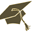Thesis (Selection of subject)(version: 356)
Assignment details
Login via CASEfektivní výpočet Coulombovských integrálů a jejich aplikace na srážkové procesy v kvantové mechanice
Thesis title in Czech: Efektivní výpočet Coulombovských integrálů a jejich aplikace na srážkové procesy v kvantové mechanice Efficient computation of Coulomb integrals and their applications to collision problems in quantum mechanics Coulomb integrals, Poisson equation, R-matrix method, numerical methods 2023/2024 Bachelor's thesis Institute of Theoretical Physics (32-UTF) Mgr. Zdeněk Mašín, Ph.D.
 Guidelines Use the R-matrix method to derive a numerical method for solving the Poisson equation by joining solutions from two parts of space. Test the approach on a simple atomic case and then extend it to a multi-center case. Compare the approach with that based on the Legendre expansion of the Coulomb interaction. Implement the new approach to ab initio R-matrix codes.
 References 1. C.A. Weatherford, E. Red, D. Joseph, and P. Hoggan, Molecular Physics 104, 1385 (2006). 2. N. Absi and P. Hoggan, International Journal of Quantum Chemistry 106, 2881 (2006). 3. Z. Mašín, J. Benda, J.D. Gorfinkiel, A.G. Harvey, and J. Tennyson, Computer Physics Communications 249, 107092 (2020).
 Preliminary scope of work in English Coulomb (or two-electron) integrals are an essential quantity needed by many computational approaches for studying multi-electron quantum mechanical systems such as molecules. These are 6-dimensional multi-center integrals describing the electron-electron interaction. In most applications the functions being integrated are Gaussians which leads to well-known analytic formulae for the Coulomb integrals. Recently, computer codes specialized to problems of collisions of electrons with molecules have switched to using numerical functions (B-splines) for the description of the unbound particle. While this opens a wide range of new problems that can be investigated it also leads to the necessity of computing a large number (billions) of mixed-basis Coulomb integrals involving Gaussians and B-splines. In our computer codes we have implemented a method based on the Legendre expansion for computation of the Coulomb integrals which has been very successful but has several limitations, especially in case of the mixed exchange integrals. In this work we will focus on development of an alternative numerical method for computation of the Coulomb integrals based on solving the Poisson equation using the R-matrix approach known from quantum mechanics. This approach should lift the limitations of the Legendre approach to the integral computation and lead to a more efficient code which will open the way to new applications. This project is flexible and can be carried out either as a bachelor or a diploma thesis.

Charles University | Information system of Charles University | http://www.cuni.cz/UKEN-329.html